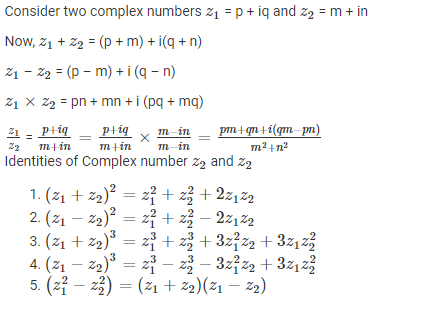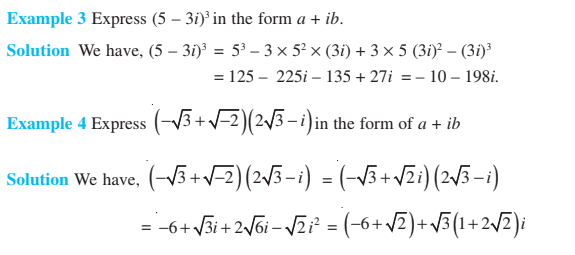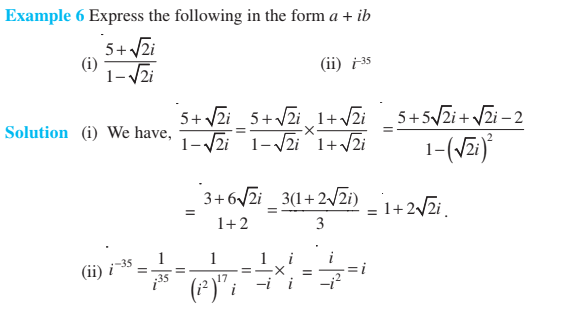# Complex Numbers Class 11

Complex Numbers Class 11 – A number that can be represented in form p + iq is defined as a complex number. [Where, p and q are real numbers and $i=\sqrt{-1}$]. For a complex number z = p + iq, p is known as the real part, represented by Re z and q is known as the imaginary part represented by Im z of complex number z.### Complex Numbers Class 11 Concepts

The topics and subtopics covered in complex numbers class 11 are:

• Introduction
• Complex Numbers
• Algebra of Complex Numbers
• Addition of two complex Numbers
• Difference of two complex Numbers
• Multiplication of two complex Numbers
• Division of two complex Numbers
• Power of i
• The square root of a negative real number
• Identities
• The Modulus and the Conjugate of a Complex Numbers
• Argand Plane and Polar Representation
• Polar Representation of a Complex Numbers

## Complex Numbers Class 11 Notes

The notes for class 11 gives a detailed knowledge of all the concepts involved in complex numbers. Some important concepts like the algebra of complex number, what is the principal argument, modulus and argument of complex numbers, and so on is given. To learn more, click here: Complex Numbers

### Complex Numbers Class 11 ExamplesStay tuned with BYJU’S – The Learning App for more Maths-related concepts and download the app to watch more engaging videos.